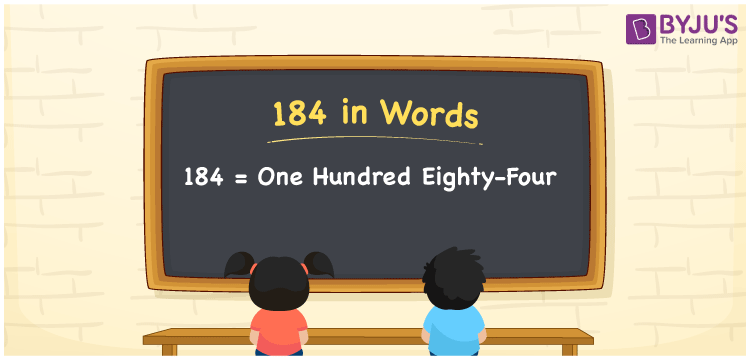# 184 in Words

184 in words is One hundred eighty-four. In other words, the number name of 184 is One hundred eighty-four. This name is used to spell or convey the number to others. For example, you paid Rs. 184 for a cold coffee at a cafe; then you can say, “I spent One hundred eighty-four rupees for the cold coffee at the cafe”. In this article, you can understand how to convert the cardinal number 184 into words and some interesting facts about 184.

 184 in words One hundred eighty-four One hundred eighty-four in Numbers 184

## 184 in English Words

Generally, we express numbers in words using the English alphabet. Thus, we spell 184 in English words as “One hundred eighty-four”.## How to Write 184 in Words?

As we can see, 184 is a three-digit number, so we need to create a three-column place value chart as shown below. Let’s see how to derive the word form of 184 using the table below.

 Hundreds Tens Ones 1 8 4

Here, ones = 4, tens = 8, hundreds = 1

By expanding these numbers, we get;

1 × Hundred + 8 × Ten + 4 × One

= 100 + 80 + 4

= One hundred + Eighty + Four

= One hundred eighty-four

Thus, 184 in words = One hundred eighty-four

As we know, 184 is a natural number that precedes 185 and succeeds 183.

184 in words – One hundred eighty-four

Is 184 an odd number? – No

Is 184 an even number? – Yes

Is 184 a prime number? – No

Is 184 a composite number? – Yes

Is 184 a perfect square number? – No

Is 184 a perfect cube number? – No

## Frequently Asked Questions on 184 in Words

Q1

### Write 184 in word form.

We can write the word form of 184 as One hundred eighty-four.
Q2

### Express 184.50 in words.

The decimal number 184.50 can be expressed in words as “One hundred eighty-four point five zero”.
Q3

### How to write Rs. 184 in words on a cheque?

On a cheque, we can write Rs. 184 in words as “One hundred eighty-four rupees only”.